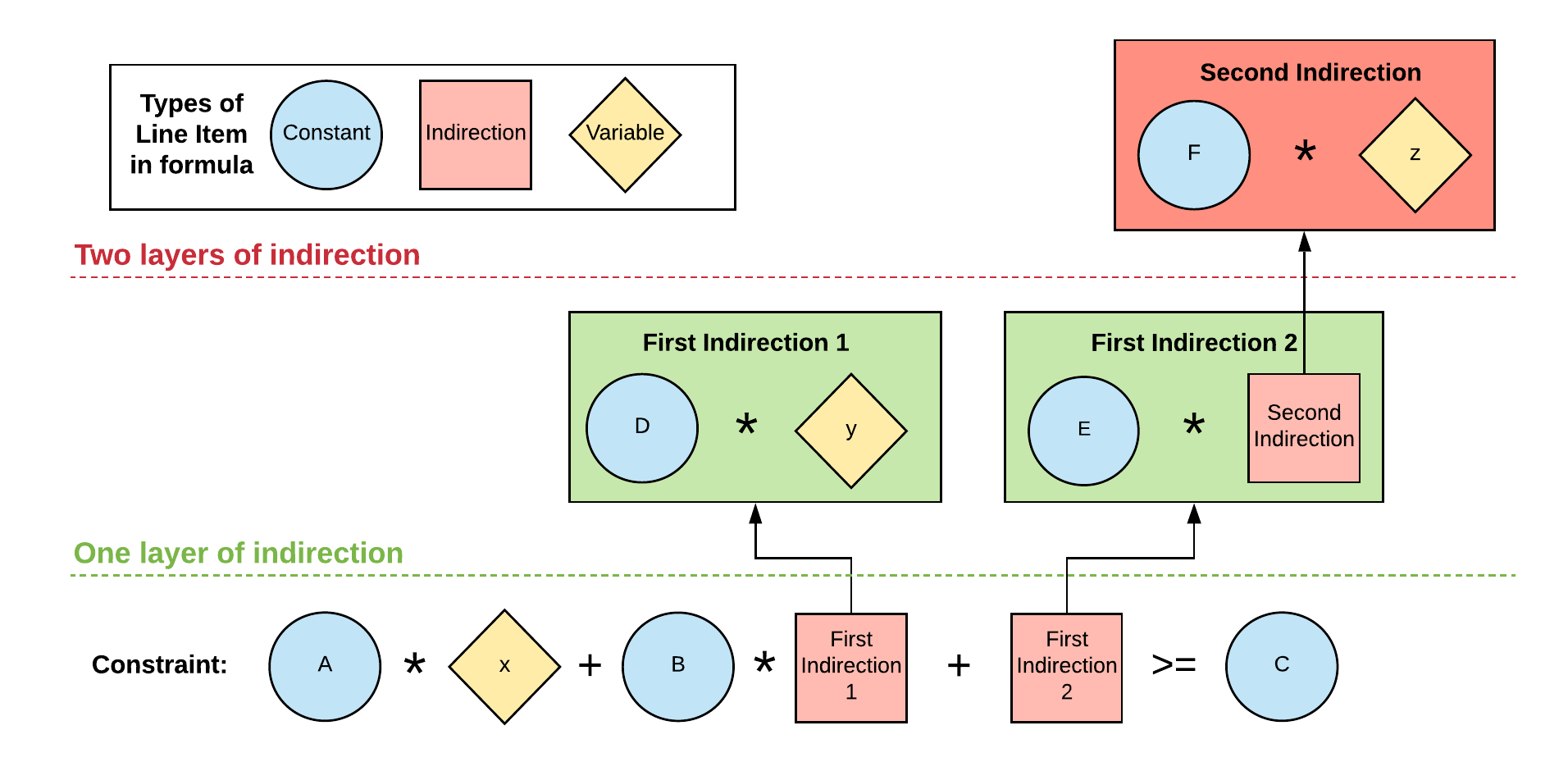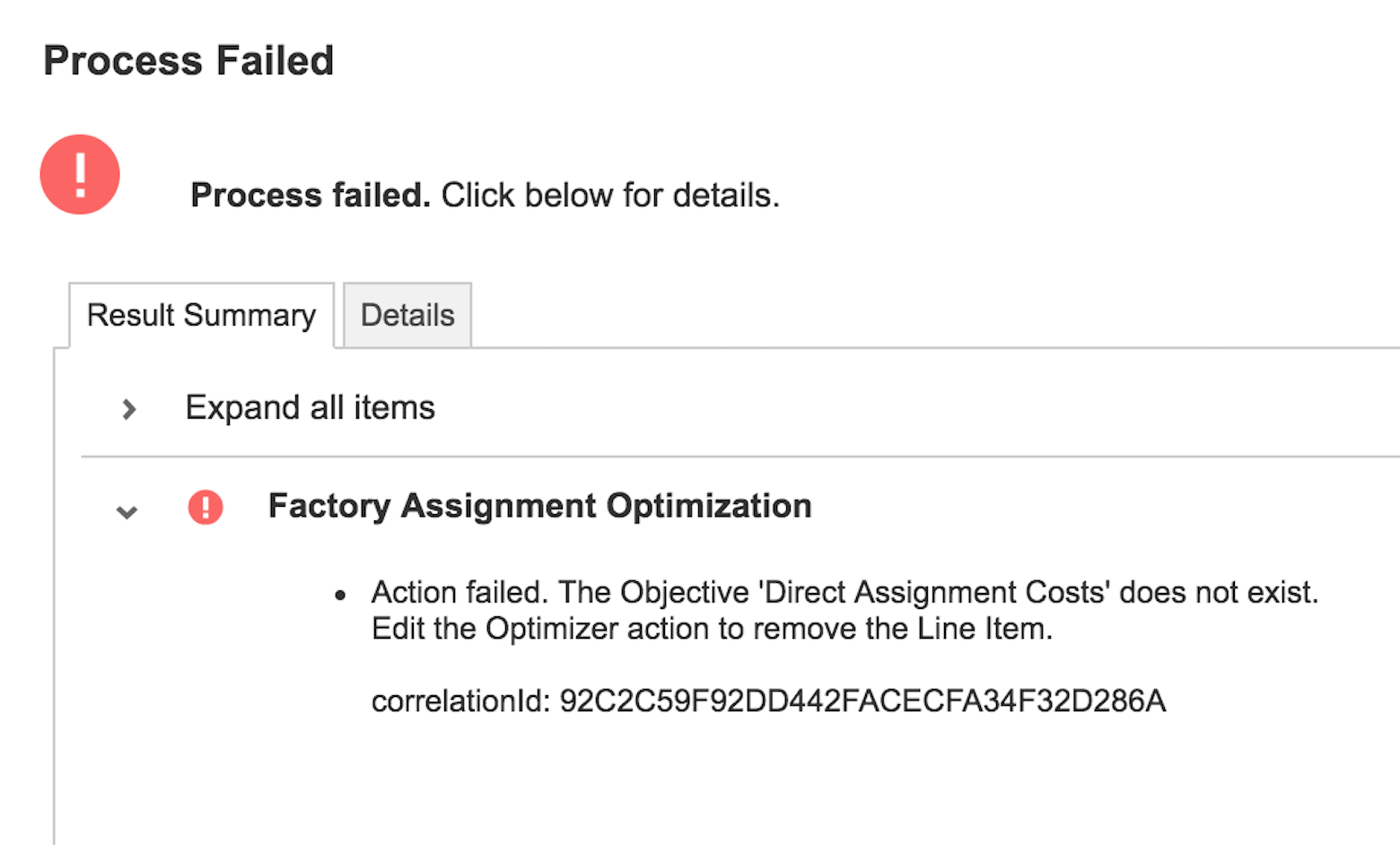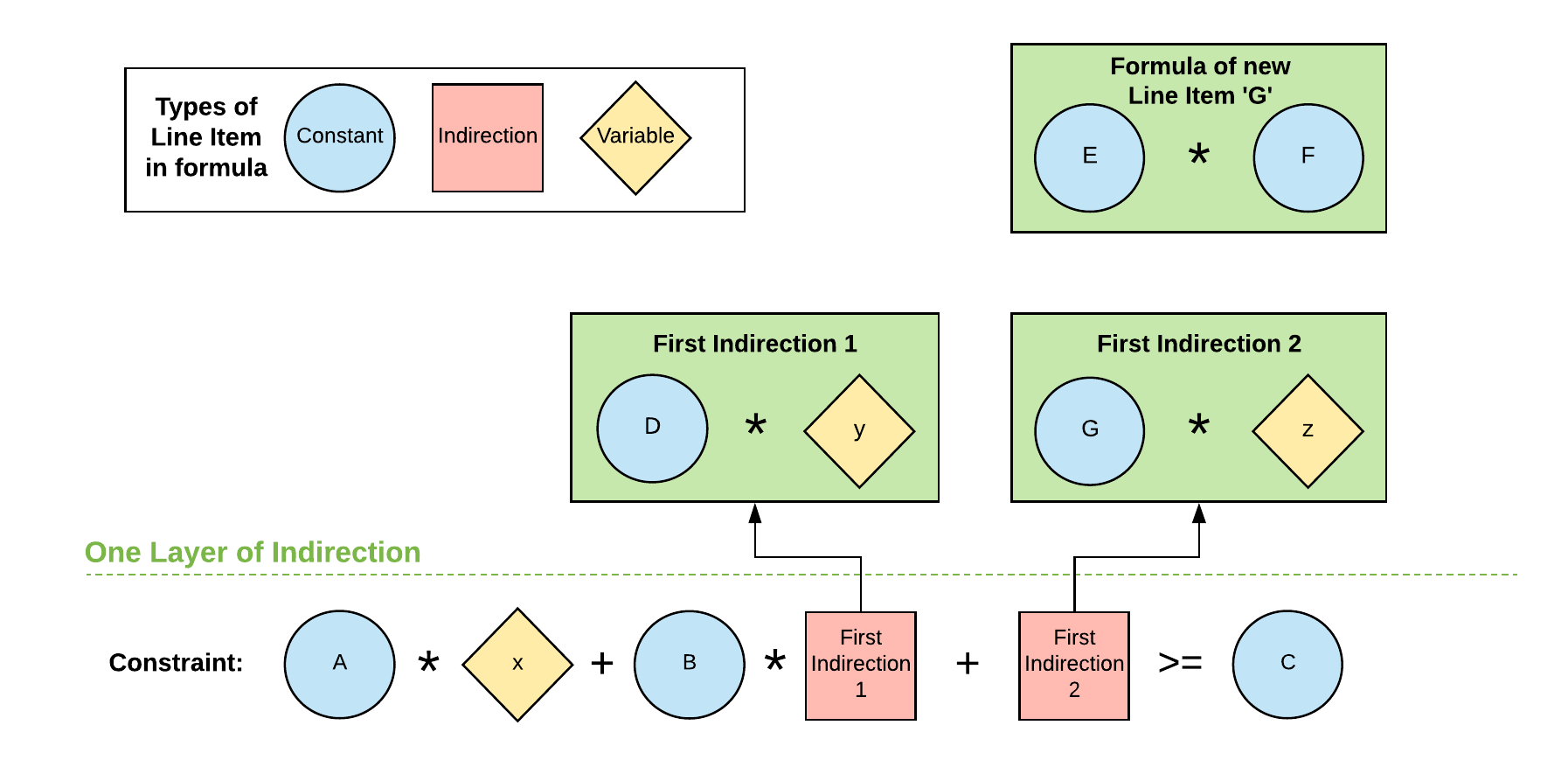1. Modeling
2. Optimizer
3. Limitations
4. Indirection

Indirection is a computer programming concept that describes a computer's ability to reference a value through a name or container, rather than the value itself. Each time that a value is referenced through such a name or container is known as a level of indirection.

In Optimizer, the line items that you use for objectives and constraints fall into one of three categories. Those that:

• are a variable;
• are dependent upon a variable; or
• are independent of variables.

Any line items that are dependent upon variables that you used in your objective or constraint formulas can only have one level of indirection (although formula components that are independent of variables can have more than one level of indirection). This means that your formulas can refer to line items that are a variable or that contain a variable — but they can't refer to line items that refer to another line item containing a variable. This concept is illustrated in the table and first diagram below.

Line Item Data Type Formula Line Item Category
x Number-formatted N/A Variable
y Number-formatted N/A Variable
z Number-formatted N/A Variable
A Number-formatted N/A Independent of variables
B Number-formatted N/A Independent of variables
C Number-formatted N/A Independent of variables
D Number-formatted N/A Independent of variables
E Number-formatted N/A Independent of variables
F Number-formatted N/A Independent of variables
G Number-formatted `E * F` Formula independent of variables
Constraint Boolean-formatted `A * x + B * First Indirection 1 + First Indirection 2 >= C` Constraint
First Indirection 1 Number-formatted `D * y` Variable-dependent formula
First Indirection 2 Number-formatted `E * Second Indirection` Variable-dependent formula
Second Indirection Number-formatted `F * z` Variable-dependent formulaIf you attempted to use the constraint formula in the diagram above in an optimization it would not work, returning an error such as the one below when you run the optimization:The error message states that an objective (or constraint) does not exist because it uses a variable with two levels of indirection, which Optimizer is not able to process.

In the diagram, the error would occur because the line item First Indirection 2 refers to a second line item Second Indirection, which contains a formula that uses a variable.

Optimization problems can be remodeled to account for the single indirection limitation. This is achieved by ensuring that any variables are referenced directly in the first level of indirection. To remodel an optimization problem:

1. Identify how any formulas in the first and second level of indirection interact with variables.
2. Isolate the variables and any attached operators; then create new line items containing the rest of the contents of these formulas.
3. Use the new line items in the first level of indirection, combining them with the variables to create new formulas that directly reference the variables.

See the diagram below for an example of how to work around the indirection limitation using the steps above.Let's break down the steps you can use to go from the first diagram to the second:

1. Identify that the indirection problem originates in Second Indirection, and by extension First Indirection 2
2. Examine the formulas used in these line items and consolidate them: `E * F * z`
3. Isolate the variable and its operator, `* z`
4. Create a new line item, G, that contains the remainder of the formula, `E * F`
5. Use the new line item G to create a new formula in First Indirection 2, `G * z`, recombining the new line item with the isolated variable and its operator.

By creating the new line item G and using it in a formula with the variable z, we are able to remove the second level of indirection. This means our constraint formula will work and the optimization can run without error.